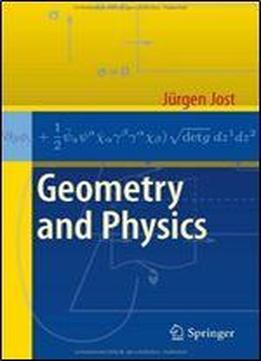# Geometry And Physics by Jurgen Jost / 2017 / English / PDF

"Geometry and Physics" addresses mathematicians wanting to understand modern physics, and physicists wanting to learn geometry. It gives an introduction to modern quantum field theory and related areas of theoretical high-energy physics from the perspective of Riemannian geometry, and an introduction to modern geometry as needed and utilized in modern physics. Jrgen Jost, a well-known research mathematician and advanced textbook author, also develops important geometric concepts and methods that can be used for the structures of physics. In particular, he discusses the Lagrangians of the standard model and its supersymmetric extensions from a geometric perspective.

views: 611